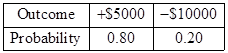# Word Problems: Expected Value

Word problems involving probability models often ask you to decide about whether an experiment is likely to turn out to your advantage or not.

Example 1:

A local club plans to invest $10000$ to host a baseball game. They expect to sell tickets worth $15000$ . But if it rains on the day of game, they won't sell any tickets and the club will lose all the money invested. If the weather forecast for the day of game is $20%$ possibility of rain, is this a good investment?

Make a table of probability distribution .Use the weighted average formula.

$\begin{array}{l}\text{Expected}\text{\hspace{0.17em}}\text{\hspace{0.17em}}\text{Value}=5000\left(0.8\right)-10000\left(0.2\right)\\ =4000-2000\\ =2000\end{array}$

The club can expect a return of $2000$ . So, it's a good investment, though a bit risky.

In other cases, we are asked to find the values of one or more variables involved in the model for which the experiment has a given expected value .

Example 2:

A company makes electronic gadgets. One out of every $50$ gadgets is faulty, but the company doesn't know which ones are faulty until a buyer complains. Suppose the company makes a $3$ profit on the sale of any working gadget, but suffers a loss of $80$ for every faulty gadget because they have to repair the unit. Check whether the company can expect a profit in the long term.

Write the probability distribution.

$\begin{array}{l}E\left(X\right)=\frac{49}{50}\cdot 3+\frac{1}{50}\cdot \left(-80\right)\\ =\frac{147}{50}-\frac{80}{50}\\ =\frac{67}{50}\\ =1.34\end{array}$

Since the expected value is positive, the company can expect to make a profit. On average, they make a profit of $1.34$ per gadget produced.